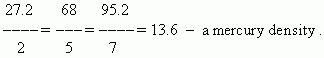# Ratio and proportion. Proportionality

Ratio. Proportion ( border and middle terms ).
The main property of a proportion.
Proportional values. Factor of a proportionality.

Ratio is a quotient of dividing one number by another.
Proportion – an equality of two ratios.
For instance:

12 : 20 = 3 : 5; a : b = c : d .

Border terms of the proportion: 12 and 5 in the first proportion;
a and d in the second proportion.

Middle terms of the proportion: 20 and 3 in the first proportion;
b and c in the second proportion.

The main property of a proportion: A product of border terms of a proportion is equal to a product of its middle terms.

Two mutually dependent values are called proportional ones, if a ratio of their values is saved as invariable. This invariable ratio of proportional values is called a factor of a proportionality .

 E x a m p l e . A mass of any substance is proportional to its volume. For instance, 2 liters of mercury weigh 27.2 kg, 5 liters weigh 68 kg, 7 liters weigh 95.2 kg. A ratio of mercury mass to its volume ( factor of a proportionality ) will be equal to:Thus, a factor of a proportionality in this example is density.